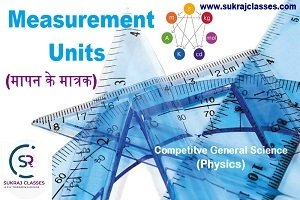# Measurement Units (मापन के मात्रक/इकाई)- Competitive Physics

Physics topic – “Measurement Units (मापन के मात्रक/इकाई)“, is important for all competitive exams like SSC CGL, SSC CHSL, RRB NTPC, UPSC and other state civil services exams. In these exams, almost 4-5 questions are coming from Physics.  Let’s start the topic – Measurement Units (मापन के मात्रक/इकाई).

## (मापन के मात्रक/इकाई)

Definition of physics: The branch of science concerned with the nature and properties of matter and energy.

Measurement:

• In Number:   1)  Octal                 2)    Decimal                      3)   Hexadecimal
• In Unit:    The word unit as used in physics binary refers to the standard measure of a quantity.

Units:     Unit are mainly two types :-

1.          Basic unit:    Total basic unit are 7  ⇒    6-physics  +   1- chemistry
2.          Derived unit:    ∞ (infinity)
• The SI base units are the standard units of measurement defined by the International System of Units(SI) for the seven base quantities.
• I. system starts in 1961 (in jenewa)
• In S.I. system dimensional formula is [M L T] where, M for mass, L for length, T for time.

S.I. System:

 Sl. No. Name of the Quantity SI Unit SI Unit Symbol 1. Length (l) Meter m 2. Mass (M) Kilogram kg 3. Time (T) Second s 4. Electric current (I) Ampere A 5. Thermodynamic temperature (Θ) Kelvin K 6. Luminous intensity (J) (light) candela cd 7 Amount of substance (N) Mole mol

This 7th unit is Chemistry unit.

Physical Quantities: – These are those quantities which can be measured accurately.

These are mainly two types:

• Scalar quantity: The physical quantity with magnitude only, no direction. E.g. Mass, Speed etc.
• Vector quantity: A vector is a quantity that has both a magnitude and a direction. For e.g.- force, velocity, acceleration, displacement, and momentum etc.

## Some Important Measurement Units of Physics:

1)  Light year:

It is unit of distance through which distance of celestial objects can be measured.

• Distance travelled by light in a year is called One-Light-year.
• Speed of light= 3 x 108­  m/sec
• Distance travelled by light in a year =  9.46  x 1012  KM    or    9.46 x 1015 meters.

2)  Parsec:

It is largest unit of distance by which distance of celestial (astronomical) object can be measured.

•   It is 3.25 times more than the light year.
•   1 parsec = 2.49 x 1013 k.m. or  2.49 x 1016 meters.

3)  Astronomical unit: –

It is also the unit of distance by which the distance of celestial (astronomical) object can be measured.

• It is average distance between Sun and Earth.
• 1-astronomical unit =  1.5 x 108 Km   or   1.5 x 1011 meters .

4)  Fermi unit: –

It is the smallest unit of distance through which atomic radius can be measured.

• It is Atomic distance between molecules.
• 1-Fermi  = 10-15  meter

5)  Nautical mile: –

This is the unit of distance through which distance in “ocean” as well as “in air” can be measure.

• 1 nautical mile = 1852 meter

6) Naute: –

It is a unit by which the speed of ship can be measured.

• naute = 1852 meter/hours

7)  Fathem: –

It is unit by which “Depth of ocean” can be measured.

• Fathem = 6 feet

8) Cusec: –

It is unit through which “flow of liquid” can be determine.

• 1 cusec = feet3 /sec

&

• 1 cusec = 28 litre

9)  Poise: –

It is a unit through which viscosity of liquid can be measured.

• Facts: “clouds” floths in the sky due to less viscosity and low density.

10)  Pascal:

It is unit through which “pressure of gas” can be measured.

• 1 pascal = 1  N/m2

11)  Diopter:   It is unit through which “power of lens” can be measure.

12)  Richter scale:Unit through which intensity of earth quack can be measured

13)  PH- Scale:   Through which “strength of acid and base” can be measured.

14)  Curine/Rutherford/Brequell: –  These are units through which “Intensity of Radio activity” can be measured.

15)  Decibel:-  It is unit through which Intensity of the sound can be measured.

16)  Aung strung (Aº):-  It is unit through which cellular component like membrane etc. can be measured.

• 1 A º= 10-10 meter